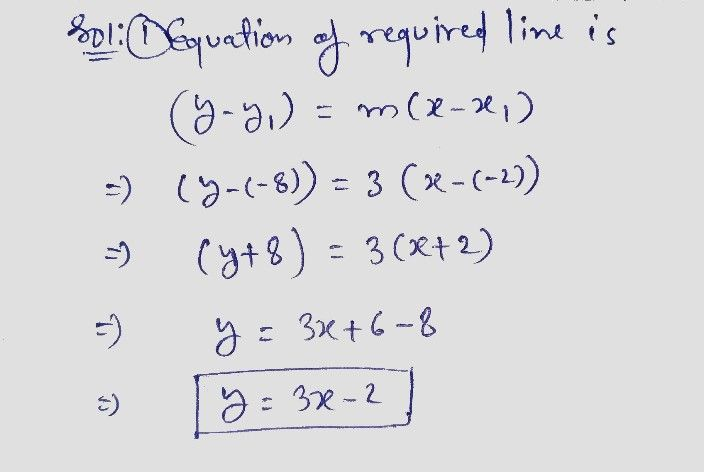Symbol
ProblemWhat is an equation of the line that passes through the point $\left(-2,-8\right)$ and has a slope of $37$ $1\right)$ $y=3x-2$ $2\right)$ $y=3x-22$ $3\right)$ $y=3x+2$ $4\right)$ $y=3x+22$